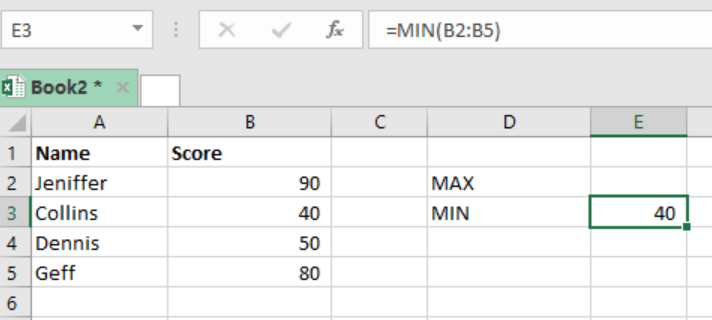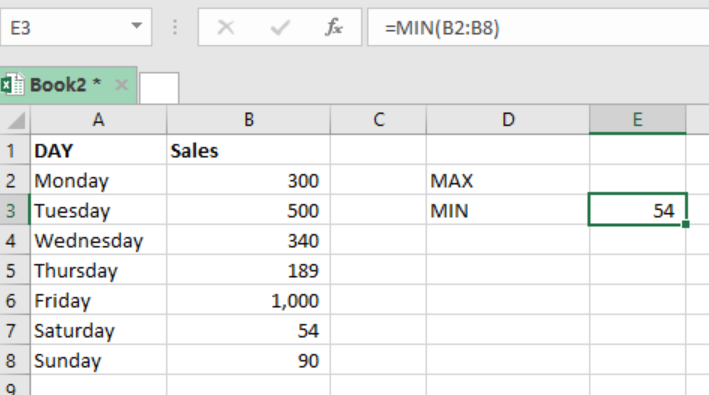Get instant live expert help with Excel or Google Sheets“My Excelchat expert helped me in less than 20 minutes, saving me what would have been 5 hours of work!”

#### Post your problem and you’ll get Expert help in seconds.

Your message must be at least 40 characters
Our professional Expert are available now. Your privacy is guaranteed.

# Excel MIN Function

If we want to find the smallest numeric value in a range of values, we can use the Excel MIN function. Here is a step-by-step guide on how to use the Excel MIN function.Figure 1: Using the Excel MIN function

## Syntax of the formula

`=MIN (number1, [number2],….)`

Where;

• Number1- refers to the numeric value or a range which contains the numeric value.
• Number2- this is optional and it refers to the numeric value or range containing the numeric value as well.

## Explanation

This function returns the smallest value within a range of numeric values. It ignores empty cells, logical values TRUE and FALSE as well as text values. But if we must include the logical arguments, then we need to use the MINA function.

The MIN function is useful when calculating fastest runner in a competition, temperatures, and lowest sales among many other things.

We can use up to 255 arguments in the formula, to find the smallest value in a given set of data. If your data has got no numbers, then the MIN function will return 0 as the smallest numeric value result.

## ExampleFigure 2: Minimum sales of the week

Here, we have used the MIN function to find the least sales of the week, which is 54 units on Saturday.

## Instant Connection to an Expert through our Excelchat Service

Most of the time, the problem you will need to solve will be more complex than a simple application of a formula or function. If you want to save hours of research and frustration, try our live Excelchat service! Our Excel Experts are available 24/7 to answer any Excel question you may have. We guarantee a connection within 30 seconds and a customized solution within 20 minutes.

### Did this post not answer your question? Get a solution from connecting with the expert.Another blog reader asked this question today on Excelchat:
Solution examplesUse the Vlookup Function to complete the "employee" column of table 2. Use "job Id" from table 2 as your lookup_value(s) and table 1 as your reference.
Solved by C. H. in 16 minsIf a cell in another sheet is populated I need a vlookup done. If the cell is not populated I need the cell to return blank.
Solved by T. D. in 60 minsI am trying to make a chart that turns a week range red if nothing is entered in the range. If something is entered then I would like it to turn green. Please Help
Solved by E. U. in 43 minsI need a check box to show/hide an answer of an if function
Solved by Z. U. in 23 minsI need a formula to compare the data in two columns and then export the mismatched data in the 3rd column
Solved by S. Q. in 20 mins## Subscribe to Excelchat.coAnother blog reader asked this question today on Excelchat: